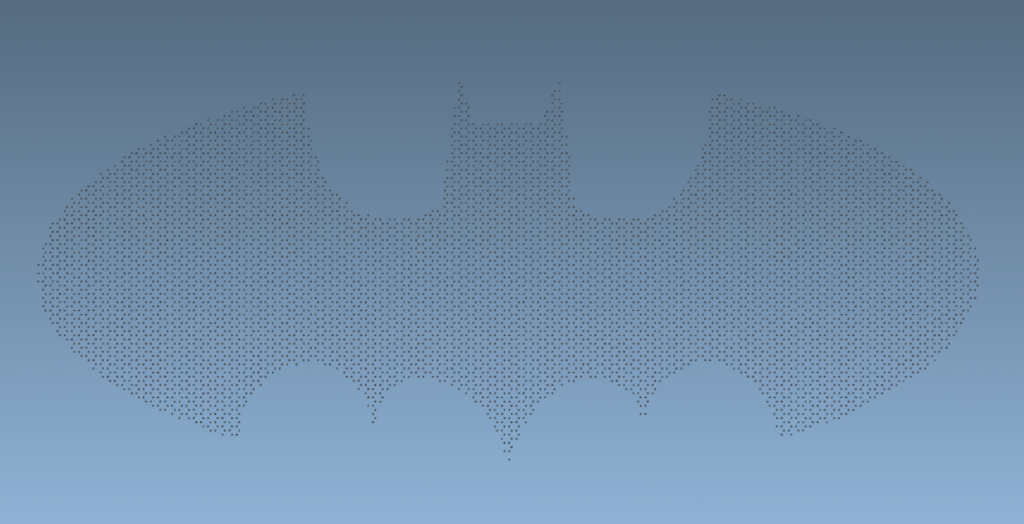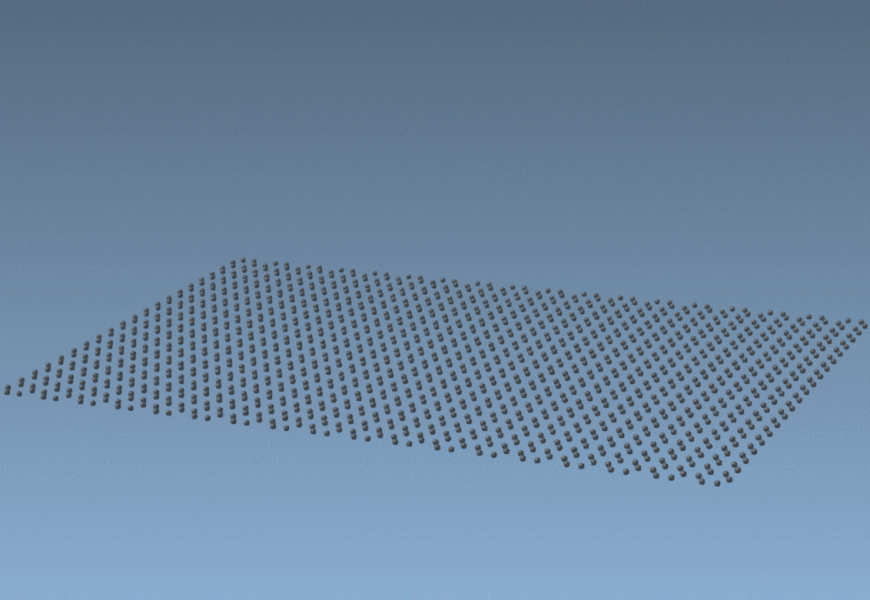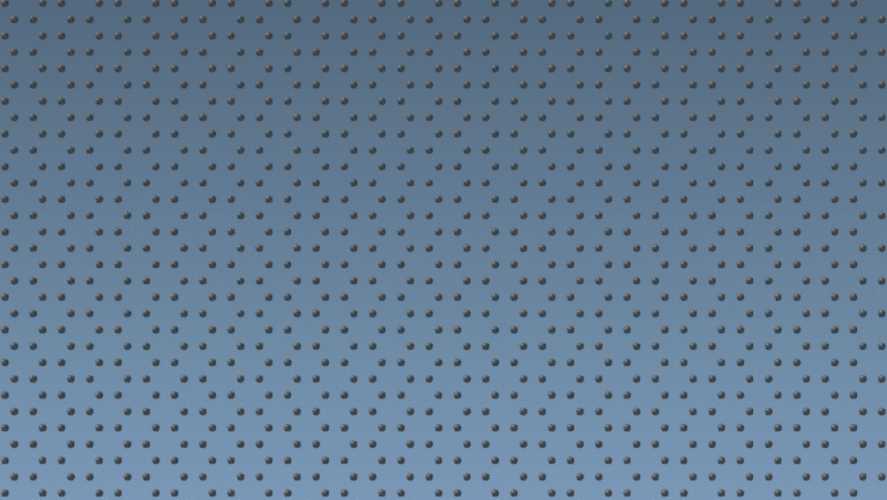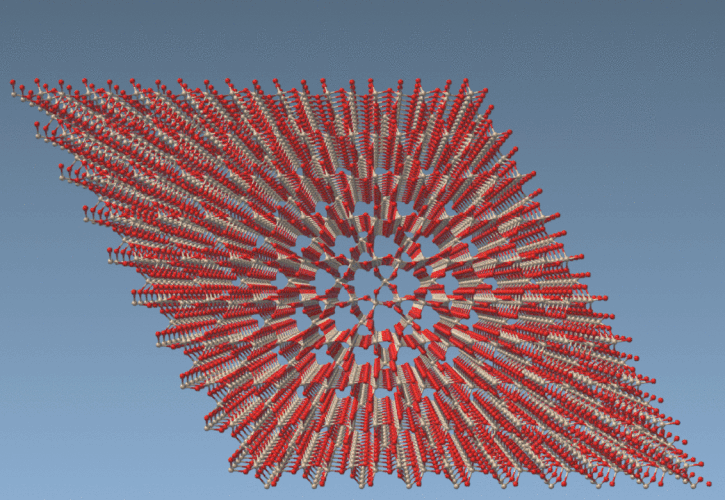# Making nano-batarangs (and more)Have you ever thought about how you could create the Batman’s batarang at the nanoscale? Maybe it won’t fight cancer or viruses, but every scientist, like a hero, needs tools to work on their cause.

In this post, we introduce two such tools that can be used for creating nano-batarangs and other complex-shaped objects at the atomic level by selecting atoms and modifying some of their properties using mathematical expressions and scripting language.

## Simple Script Extension

The Simple Script Extension allows you to modify some properties of atoms (positions, visibility, element type, etc) in the active document according to a script provided by you. To do so, predefined variables, mathematical and logical expressions can be used in the script. Below is a description of the App’s functionality.

Content:
I. Variables
II. Capabilities: operations, functions, structures
III. Examples

## I. Variables

Variables are defined as in the SAMSON Node Specification Language, but only for atoms. The difference of this Extension’s scripting language with the Node Specification Language is that all characters (i.e., names and symbols for elements) should be used within single quotation marks, e.g.: ‘Carbon’, ‘Fe’. Below is a list of variables that are possible to use in the script.

### Node attributes:

• n.selectionFlag (short name n.sf) – true/1 if node is selected, false/0 if node is not selected

### Atom attributes:

• a.x, a.y, a.z – atom’s positions
• a.visibilityFlag (short name a.vf) – true/1 if atom is visible, false/0 if atom is invisible
• a.aminoAcidBackbone (short name a.aabb) – true/1 if atom belongs to an amino-acid backbone
• a.aromatic (short name a.ar) – true/1 if atom is aromatic
• a.chainID (short name a.ci) – index of a chain to which atoms belongs to (a.ci==0 matches atoms from chain ID 0)
• a.formalCharge (short name a.fc) – atom’s formal charge
• a.nucleicAcidBackbone (short name a.nabb) – true/1 if atom belongs to a nucleic-acid backbone
• a.occupancy (short name a.oc) – atom’s occupancy
• a.partialCharge (short name a.pc) – atom’s partial charge
• a.residueSequenceNumber (short name a.resi) – index of a residue to which atom belongs to (a.resi==12 matches atoms in residue 12)
• a.resonance (short name a.reso) – true/1 if atom is resonant
• a.serialNumber (short name a.sn) – atom’s serial number (a.sn>=500 matches atoms with serial number larger than 500)
• a.temperatureFactor (short name a.tf) – atom’s temperature factor
• a.water (short name a.w) – true/1 if atom belongs to water molecule
• a.element (short name a.e) – element name of atom (a.e=='Carbon' matches carbon atoms)
• a.symbol (short name a.s) – element symbol of atom (a.s=='H' matches hydrogen atoms)
• a.elementID (short name a.ei) – element index of atom in the periodic table
• a.vdwr – van der Waals radius of atom

It is possible to change only the next properties of atoms: n.selectionFlag, a.x, a.y, a.z, a.visibilityFlag, a.chainID, a.formalCharge, a.occupancy, a.partialCharge, a.serialNumber, a.temperatureFactor, a.elementID.

## II. Capabilities: operators, functions, structures

It is possible to use standard mathematical operators and functions, and C-like structures and statements.

1. Basic operators: +, -, *, /, %, ^
2. Assignment: :=, +=, -=, *=, /=, %=
3. Equalities and inequalities: =, ==, <>, !=, <, <=, >, >=
4. Logic operators: and, mand, mor, nand, nor, not, or, shl, shr, xnor, xor, true, false
5. Functions: abs, avg, ceil, clamp, equal, erf, erfc, exp, expm1, floor, frac, log, log10, log1p, log2, logn, max, min, mul, ncdf, nequal, root, round, roundn, sgn, sqrt, sum, swap, trunc
6. Trigonometry: acos, acosh, asin, asinh, atan, atanh, atan2, cos, cosh, cot, csc, sec, sin, sinc, sinh, tan, tanh, hypot, rad2deg, deg2grad, deg2rad, grad2deg
7. Control structures: if-then-else, ternary conditional, switch-case, return-statement
8. Loop statements: while, for, repeat-until, break, continue
```# this is a comment // another comment /* this is also a comment */```

Parsing and evaluation of expressions are done thanks to the C++ Mathematical Expression Parsing And Evaluation Library ‘exprtk’ by Arash Partow. See the list of possible operators, structures and other capabilities here: exprtk by Arash Partow.

Note: all variable and function names are case-insensitive.

## III. Examples:

In this section, a brief introduction to the scripting language is given in several examples.

1. Set z-coordinate equal to sin(a.x * π / 12) for all atoms in the active document:
`a.z:=sin(a.x * pi / 12);`2. Shift all atoms with type 2 in y-direction by 5.25 (in the selected length units):
`if (a.elementID==2) a.y+=5.25;`

or the same expression using short names:

`if (a.ei==2) a.y+=5.25;`
3. Select all atoms with x and y coordinates greater than 0:
```if (a.x &gt; 0 and a.y &gt; 0) n.selectionFlag:=1; else n.selectionFlag:=0;```

or the same using ternary conditional statement:

`( (a.x &gt; 0) and (a.y &gt; 0) ) ? n.sf:=1 : n.sf:=0;`
4. Select all Carbon and Hydrogen atoms:
```if (a.element=='Carbon' or a.symbol=='H') n.selectionFlag:=1; else n.selectionFlag:=0;```
5. Show only atoms inside a sphere with radius 10 and center in (0, 0, 2) (in the selected length units):
```if ((a.x^2 + a.y^2 + (a.z - 2)^2) &lt; 100) a.visibilityFlag:=1; else a.visibilityFlag:=0;```
6. Change elementID of atoms from 1 to 2 (works only with a.elementID):
`if (a.elementID==1) a.elementID:=2;`
7. Shift atoms with elementID equal to 1 an with x-coordinate |x|>10 by 5 in the y-direction (in the selected length units):
`if (a.ei==1 and abs(a.x) &gt; 10) a.y+=5;`
8. Rotate all atoms about the x-axis by an angle θ=π/4:
```var theta:=pi/4; # create variable, the same as: theta:=deg2rad(45); var y_old:=a.y; var z_old:=a.z; a.y:=y_old * cos(theta) - z_old * sin(theta); a.z:=y_old * sin(theta) + z_old * cos(theta);```
9. Switch operator:
```switch { case a.x &lt; -1 : a.y += 1.5; case a.x &gt; 1 : a.y -= 1.5;   default : a.y:=a.y; };```
10. Modify z-coordinate for atoms with |x|>10 and highlight them:
```if ( abs(a.x) &gt; 10 ) {   n.sf := 1;   a.z := sin( a.x * pi / 12); } else {   n.sf:=0; };```
11. Example on nested for-loops:
```for (var i:=0; i &lt; 4; i+=1) {   a.y -= 1;   if (a.y &lt; 0)     continue;   for (var j := 0; j &lt; 4; j += 1) {   a.z += 2;     if (a.z &gt; 10)       break;   }; };```
12. This scripting language can be used for complex selection of atoms in the active document. For example, one can cut nano-tiles from graphene:13. And, finally, one can create Batman’s nano-batarang out of graphene.Script for creating the nano-batarang out of graphene sheet placed in the z-plane:

```/* Batman's sign */ var x:=a.x / 2; // positions should be at least in nanometers var y:=a.y / 2; var iambatman:=false; if ( (abs(x)&gt;3 and y&gt;=0) or (abs(x)&gt;4 and y&lt;0)){ // wings, ellipsoidal sides if( (x/7)^2+(y/3)^2 &lt; 1 ) iambatman:=true; } else if (abs(x)&lt;=4 and y&lt;0) { // central bottom part if (y &gt;= (abs(x/2) - (3*sqrt(33)-7)*x^2/112 - 3) + sqrt(1-(abs(abs(x)-2)-1)^2) ) iambatman:=true; } else if (abs(x)&lt;=1 and abs(x)&gt;0.75 and y&gt;=0) { // sides of the head if (y &lt;= 9 - 8*abs(x) ) iambatman:=true; } else if (abs(x)&lt;=0.75 and abs(x)&gt;0.5 and y&gt;=0) { // pointy ears if (y &lt;= 3*abs(x) + 0.75 ) iambatman:=true; } else if (abs(x)&lt;=0.5 and y&gt;=0) { // top of the head if (y &lt;= 2.25) iambatman:=true; } else if (abs(x)&lt;=3 and abs(x)&gt;1 and y&gt;=0) { // from head to wings if (y &lt;= 6*sqrt(10)/7 + (1.5-0.5*abs(x)) - 6*sqrt(10)/14*sqrt(4-(abs(x)-1)^2)) iambatman:=true; }; if (iambatman) { // set selectionFlag and visibilityFlag to 1 if atom is selected n.sf:=1; a.vf:=1; } else { // set selectionFlag and visibilityFlag to 0 if atom is not selected n.sf:=0; a.vf:=0; };```
14. Let’s now use switch instead of multiple if-statements to create a graphene batarang from Nolan’s trilogy.Script for creating the nano-batarang out of graphene sheet placed in the z-plane:

```/* Batarang from Nolan's trilogy */ var x:=a.x / 2; // positions should be at least in nanometers var y:=a.y / 2; var iambatman:=false; if (y &gt;= 0 and y &lt;= 3) { switch { // wings, top case (abs(x)&gt;4 and abs(x)&lt;10) : if( ((abs(x)-14)/4)^2+((y+2)/3)^2 &gt; 4 ) { iambatman:=true; }; // from head to wings case (abs(x)&gt;1 and abs(x)&lt;=4) : if( ((abs(x)-1)/1.75)^2+(y-3)^2 &gt; 3 ) { iambatman:=true; }; // sides of the head case (abs(x)&lt;=1 and abs(x)&gt;0.75) : if( y &lt;= 9 - 8*abs(x) ) { iambatman:=true; }; // pointy ears case (abs(x)&lt;=0.75 and abs(x)&gt;0.5) : if( y &lt;= 3*abs(x) + 0.75 ) { iambatman:=true; }; // top of the head case abs(x)&lt;=0.5 : if( y &lt;= 2.25 ) { iambatman:=true; }; default : iambatman:=false; }; } else if (y &lt; 0 and y &gt; -3.5){ switch { case (abs(x)&lt;=6) : if( ((abs(x)-8)/4)^2+((y+3.5)/1.5)^2&gt;4 ) { iambatman:=true; }; case (abs(x)&lt;=10 and abs(x)&gt;6) : if( ((abs(x)-14)/4)^2+((y+2)/3)^2&gt;4 and y&gt;-0.5 ) { iambatman:=true; }; default : iambatman:=false; }; } if (iambatman) { // set selectionFlag and visibilityFlag to 1 if atom is selected n.sf:=1; a.vf:=1; } else { // set selectionFlag and visibilityFlag to 0 if atom is not selected n.sf:=0; a.vf:=0; };```

## Atoms Selector Extension

If you want to just select atoms using a mathematical expression, the Atoms Selector Extension might be of help. The same variables, operators and functions as in the Simple Script Extension can be used. In Atoms Selector Extension, you only need to provide an expression according to which you want atoms in the active document to be selected.

An additional keyword can be used:

• all – select all atoms [in the active document].

For example, to cut a cylinder with radius 10Å out of quartz crystal (given known positions of the crystal):

`((a.x-15)^2 + (a.y-15)^2) &lt; 100`Please, let us know in the comments below what you’d like to see in SAMSON or if you have any questions.

And, to paraphrase Bruce Wayne, an App developer can be anyone! You can develop your own Apps for your needs in SAMSON thanks to the powerful SAMSON SDK.

If you have any questions or feedback, please use the SAMSON forum.